Word Explorer Children's Dictionary

Display options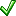Show syllablesShow Lookup HistoryDouble-Click LookupShow Word Parts
Show Spanish support
Show Chinese support

PronunciationWordsmyth Phonics IPA

# num·ber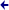# number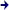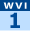pronunciation:
nuhm b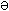r
parts of speech:
noun, verb
features:
Word Explorer
 part of speech: noun definition 1: a unit in math with a fixed value that is used in counting or to tell the position of something in a series. "Four," "four tenths," and "four hundred" are all ways of saying numbers. Numbers can also be represented by numerals, such as 4, 0.4, and 400. We use numbers when we add, subtract, multiply, or divide.   similar words: cardinal, digit, figure, integer, numeral definition 2: a numeral or symbol that stands for a number. Football players wear numbers on their shirts.synonyms: figure, numeral similar words: character, digit, symbol definition 3: a number or numeral used to label or name something. Write down my telephone number.similar words: numeral definition 4: total amount; sum. The number of people in this room is eight.synonyms: amount, sum similar words: figure, tally, total definition 5: a quantity that is equal to at least a few and perhaps more. A number of icicles formed on the roof.synonyms: amount, quantity similar words: abundance, army, crowd, host, multitude definition 6: one piece of a musical program. The pianist played a lively number.similar words: composition, piece, routine, sketch, song related words: dance, many, volume part of speech: verb inflections: numbers, numbering, numbered definition 1: to set apart or label with a number. Please number your pages. definition 2: to limit in number or amount. The sheriff told the outlaw that his days of stealing horses were numbered.similar words: confine, limit, restrict definition 3: to exist in a certain amount. These snakes number in the thousands in this region.similar words: amount to, total definition 4: to amount to a total of. Guests at the party numbered twenty.synonyms: total definition 5: to be included. She numbers as one of the few students who won a prize in the writing contest.similar words: belong related words: add derivation: numbered (adj.)
Word Explorer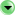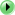a broader category that includes numbers mathematicssimilar to a number numeral, symbolsome actions associated with numbers add, average, average out, calculate, compute, count, divide, estimate, figure, measure, multiply, round, square, subtractsome descriptions of numbers binary, even, irrational, odd, primesome kinds of numbers Arabic numeral, cardinal number, decimal, fraction, improper fraction, integer, mixed number, ordinal number, power, Roman numeral, root, whole numbersome parts of numbers billions, billionths, decimal point, digits, hundreds, hundredths, millions, millionths, thousandssome people who frequently use numbers accountant, architect, astronaut, clerk, engineer, mathematician, navigator, pilot, scientistsome qualities of numbers equal, infinite, large, maximum, minimum, negative, positive, small, unequal, zerosome things that contain or use numbers address, area code, calendar, combination, coordinate, countdown, dice, grade, index, telephone, zip code